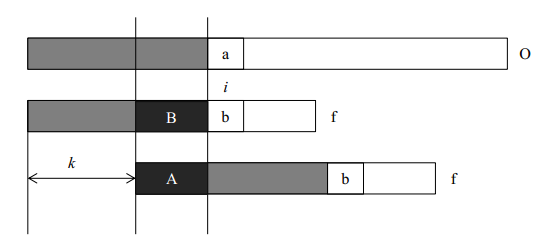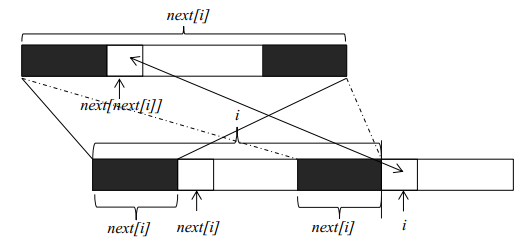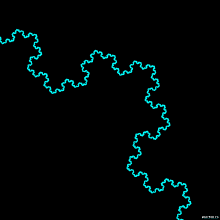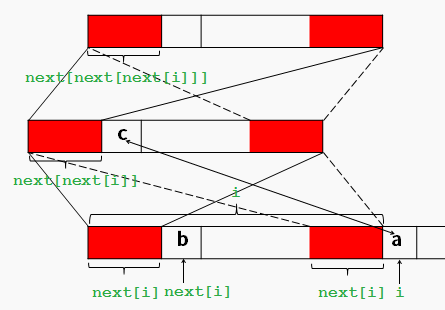KMP算法学习(详解)

kmp算法又称“看毛片”算法，是一个效率非常高的字符串匹配算法。不过由于其难以理解，所以在很长的一段时间内一直没有搞懂。虽然网上有很多资料，但是鲜见好的博客能简单明了地将其讲清楚。在此，综合网上比较好的几个博客（参见最后），尽自己的努力争取将kmp算法思想和实现讲清楚。

kmp算法完成的任务是：给定两个字符串O和f，长度分别为n和m，判断f是否在O中出现，如果出现则返回出现的位置。常规方法是遍历a的每一个位置，然后从该位置开始和b进行匹配，但是这种方法的复杂度是O(nm)。kmp算法通过一个O(m)的预处理，使匹配的复杂度降为O(n+m)。

kmp算法思想

• A段字符串是f的一个前缀。
• B段字符串是f的一个后缀。
• A段字符串和B段字符串相等。next数组计算

1 public int[] getNext(String b)
2 {
3     int len=b.length();
4     int j=0;
5
6     int next[]=new int[len+1];//next表示长度为i的字符串前缀和后缀的最长公共部分，从1开始
7     next=next=0;
8
9     for(int i=1;i<len;i++)//i表示字符串的下标，从0开始
10     {//j在每次循环开始都表示next[i]的值，同时也表示需要比较的下一个位置
11         while(j>0&&b.charAt(i)!=b.charAt(j))j=next[j];
12         if(b.charAt(i)==b.charAt(j))j++;
13         next[i+1]=j;
14     }
15
16     return next;
17 }

字符串匹配

1 public void search(String original, String find, int next[]) {
2     int j = 0;
3     for (int i = 0; i < original.length(); i++) {
4         while (j > 0 && original.charAt(i) != find.charAt(j))
5             j = next[j];
6         if (original.charAt(i) == find.charAt(j))
7             j++;
8         if (j == find.length()) {
9             System.out.println("find at position " + (i - j));
10             System.out.println(original.subSequence(i - j + 1, i + 1));
11             j = next[j];
12         }
13     }
14 }

复杂度

kmp算法的复杂度是O(n+m)，可以采用均摊分析来解答，具体可参考算法导论。

1.     kmp算法小结

2.     kmp算法详解

3.     kmp算法

PS：posted @ 2017-04-23 07:59  Angel_Kitty  阅读(8923)  评论(11编辑  收藏  举报